# Revision on Fractions Primary 4 (Basic 4) Term 2 Week 1 Mathematics

### MATHEMATICS

SECOND TERM

WEEK 1

PRIMARY 4

THEME – NUMBER AND NUMERATION

PREVIOUS LESSON – First Term Examination Mathematics Primary 4 (Basic 4) – Exam Questions

### LEARNING AREA

1. Introduction

2. Meaning and Types of Fractions

3. Relationships between Improper and Mixed Fractions and Vice Versa

4. Revision Test

### LEARNING OBJECTIVES

By the end of the lesson, most pupils should have attained the following objectives –

1. explain the meaning of fraction.

2. identify each type of fraction.

3. differentiate between proper and improper fractions.

4. change improper fractions to mixed fractions and vice versa.

5. attempt most of the revision test correctly.

### ENTRY BEHAVIOUR

The pupils can share fruits, bread, etc. equally or more than one another.

### INSTRUCTIONAL MATERIALS

The teacher will teach the lesson with the aid of:

1. Orange

2. Paper cuttings of different shapes

3. Fraction chart

4. Squares

5. Cardboards

6. Flow chart of quantitative reasoning

7. Flash cards

### METHOD OF TEACHING

Choose a suitable and appropriate methods for the lessons.

Note – Irrespective of choosing methods of teaching, always introduce an activities that will arouse pupil’s interest or lead them to the lessons.

### REFERENCE MATERIALS

1. Scheme of Work

2. 9 – Years Basic Education Curriculum

3. Course Book

4. All Relevant Material

5. Online Information

### LESSON 1 – INTRODUCTION

ACTIVITY 1 – PARTS OF WHOLEA wholeEqual PartsOne Part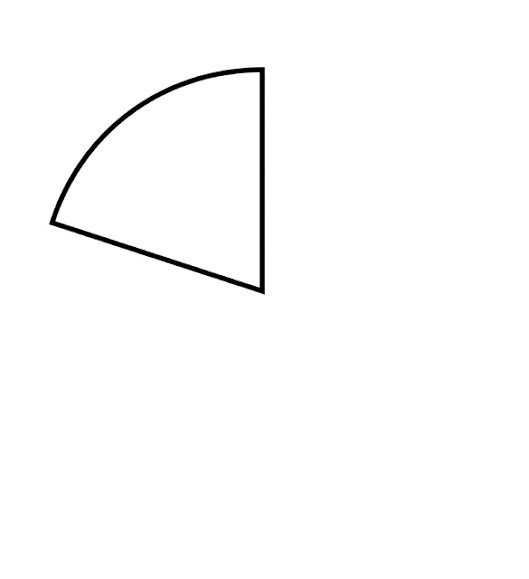One part or one – fifth

One fifth means one part in five equal parts.Two parts or Two – fifth

Two fifth means two parts in five equal parts.Three parts – Three – fifth

Three fifth means three parts in five equal parts.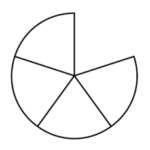Four – fifth

Fourfifth means four parts in five equal parts.

ACTIVITY 2 – MEANING OF FRACTION

A part of a whole is called fraction.

Fraction can be defined as a part or parts of a whole.

ACTIVITY 3 – NUMERATOR AND DENOMINATOR

A fraction has two parts. That’s, the numerator and denominator.

For example, in activity 1

One – fifths is written 1/5.

Two – fifths is written as 2/5.

Three – fifth is written as 3/5.

Four – fifth is written as 4/5.

Fifth – fifth is written as 5/5.

### DENOMINATOR

We call the top number the Numerator, it is the number of parts we have.

We call the bottom number the Denominator, it is the number of parts the whole is divided into.

ACTIVITY 4 – LESSON EVALUATION

1. Fraction is a part of a ______.

A. whole

B. part

C. all

2. What is a fraction?

A. A fraction is a whole of a part.

B. A fraction is part of a whole.

C. A fraction is a part and a whole.

Use the following shapes for questions 3 – 6.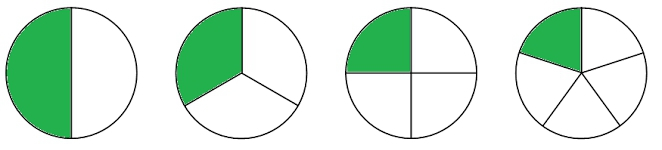### A             B               C               D

3. What is the fraction of shaded part of A?

4. The unshaded part of C is ______.

6. What is the denominator of unshaded part of D?

7. ______ is the number of parts we have.

A. Numerator

B. Denominator

8. ______ is the number of parts the whole is divided into.

A. Numerator

B. Denominator

9. Four ninth means ______.

A. four parts in nine equal parts.

B. nine parts in four equal parts.

10. An object is divided into 10 equal parts. Three parts is called ______.

A. 10/3

B. 3/10

C. 3-10

1. A – whole

2. B – A fraction is part of a whole.

3. Half or 1/2

4. 2/3 or two – third

5. 1/3 and 4/5

6. 5

7. A – Numerator

8. B – Denominator

9. A. four parts in nine equal parts.

10. B. 3/10

### LESSON 2 – TYPES OF FRACTIONS

ACTIVITY 1 – REVISION OF LESSON 1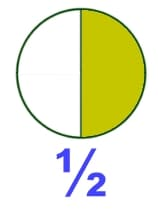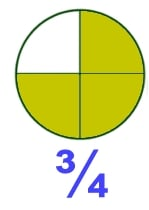ACTIVITY 2 – COMPARING NUMERATOR AND DENOMINATOR

A.

# 2/5

Numerator is 2 while denominator is 5.

The numerator is less than denominator,

B.

# 7/5

Numerator is 7 while denominator is 5.

The denominator is greater than numerator,

# 7 > 5

QUICK EVALUATION

1. State 3 examples of fractions with each numerator greater than denominator.

2. Give 3 examples of fractions with each denominator greater than numerator.

ACTIVITY 3 – PROPER AND IMPROPER FRACTIONS

2/5 is a proper fraction

while

7/5 is an improper fraction.

Note – Both fractions have the same denominator but their numerators are different. One has smaller numerator than denominator while the other one has numerator greater than denominator.

A proper fraction is a fraction where numerator is less than the denominator.

### 5 – greater

An improper Fraction is a fraction where denominator is greater than numerator.

### 5 – smaller

ACTIVITY 4 – MIXED FRACTIONS

Share the following burger among two pupils.

# 🌭🌭🌭🌭🌭

Each of them will get two and share the last one into two equal parts.

# 🌭🌭     🌭    🌭🌭

Each one of them with get two and a half.

Two and a half is written as 2½.

# 2½

2½ stands for whole plus a fraction.

2 is a whole number ½ a fraction.

This kind of fraction is known as mixed fraction.

A mixed fraction is the combination of a whole number and a fraction.

ACTIVITY 5 – TYPES OF FRACTIONS

There are three major types of fractions.

1. Proper fractions

2. Improper fractions

3. Mixed fractions

ACTIVITY 6 – LESSON EVALUATION

1. Fraction is a part of a ______.

A. whole

B. part

C. all

2. What is a fraction?

A. A fraction is a whole of a part.

B. A fraction is a part of a whole.

C. A fraction is a part and a whole.

3. There are ______ types of fractions.

A. Thirty

B. Three

C. Third

4. ______ is a fraction where numerator is less than the denominator.

A. Mixed fraction

B. Proper fractions

C. Improper fraction

5. ______ is a fraction where denominator is greater than numerator.

A. Mixed fraction

B. Proper fraction

C. Improper fraction

6. ______ is the combination of a whole number and a fraction.

A. Mixed fraction

B. Proper fraction

C. Improper fraction

7. 5¾ is a good example of ______.

A. Mixed fraction

B. Proper fraction

C. Improper fraction

8. 8/5 is a good example of ______.

A. Mixed fraction

B. Proper fractions

C. Improper fraction

9. 5/8 is a good example of ______.

A. Mixed fraction

B. Proper fractions

C. Improper fraction

10. Differentiate between the following –

A. Proper and improper fractions

B. Mixed fractions and other types of fractions

C. What is the similarity between proper and mixed fractions.

1. A – whole

2. B – A fraction is a part of a whole.

3. B – Three

4. B – Proper fraction

5. C – Improper fraction

6. A – Mixed fraction

7. A – Mixed fraction

8. C – Improper fraction

9. B – Proper fraction

10. Teacher’s discretion

### LESSON 3 – RELATIONSHIP BETWEEN PROPER AND MIXED FRACTIONS

ACTIVITY 1 – REVISION OF THE PREVIOUS LESSONS

A fraction is a part of a whole.

There are 3 types of fractions.

1. Proper fractions

2. Improper fractions

3. Mixed fractions

A proper fraction is a fraction where numerator is less than the denominator.

### 5 – greater

Note – Proper fractions are also fractions less than one.

An improper Fraction is a fraction where denominator is greater than numerator.

### 5 – smaller

Note – Improper fractions are also fractions greater than one.

A mixed fraction is the combination of a whole number and a fraction.

# 2¾

ACTIVITY 2 – CHANGING IMPROPER FRACTIONS TO MIXED FRACTIONS

Examples of improper fractions are –

# 11/5, 7/2, 9/7

Each of the following can be expressed as

= 1 + 1 + 1/6

= 2 + 1/6

= 2 1/6

= 3 1/2

### 7

= 1 2/7

ACTIVITY 3 – FURTHER EXAMPLES

1. 25/7

7 + 7 + 7 + 4

________________

7

= 3 4/7

2. 30/11

11 + 11 + 8

________________

11

= 2 8/11

3. 37/5

5 + 5 + 5 + 5 + 5 + 5 + 5 + 2

________________________________

5

= 7 2/5

ACTIVITY 4 – LESSON EVALUATION

1. _____ is greater than 1.

A. Proper fraction

B. Improper fraction

C. Both

2. Which of these is less than 1?

A. Proper fraction

B. Improper fraction

C. Mixed fraction

3. _____ can be expressed as mixed fractions.

A. Proper fractions

B. Improper fractions

C. Proper and improper fractions

4. Which of these fractions cannot be expressed as mixed fractions?

A. Proper fraction

B. Improper fraction

C. Both

Change the following mixed fractions.

5. 15/4

6. 21/2

7. 41/12

8. The following are mixed fractions except.

A. 2 3/4

B. 2 4/3

C. 3 3/4

9. 15/ 2 is

A. 7 1/2

B. 7 2/1

C. 7 1/12

10. 41/12 = 3 5/12

A. False

B. True

C. None of the above

1. B – improper fraction

2. A – Proper fraction

3. B. Improper fraction

4. A Proper fraction

5. 15/4

=     4 + 4 + 4 + 3

_______________

4

= 3 3/4

6. 21/2

=     2 + 2 + 2 + 2 + 2 + 2 + 2 + 2 + 2 + 2 + 1

_____________________________________

2

= 10 1/2

7. 41/12

=     12 + 12 + 12 + 5

_______________

12

= 3 5/12

8. B – 2 4/3

9. A – 7 1/2

10. B – True

### LESSON 4 – MIXED FRACTIONS AND IMPROPER FRACTIONS

ACTIVITY 1 – REVISION OF LESSON 3

As stated in lesson 3 evaluation.

ACTIVITY 2 – MIXED FRACTIONS

A mixed fraction is the combination of a whole number and a fraction.

The following are examples of mixed fractions –

1. 3 5/12

2. 10 1/12

3. 7 2/5

Note – Improper fractions can be changed to mixed fractions. Likewise, mixed fractions can also be changed to improper fractions.

ACTIVITY 3 – CHANGING MIXED FRACTIONS TO IMPROPER FRACTIONS

1. 3 5/12

=      12 x 3 + 5

______________

12

=      36 + 5

___________

12

= 41/12

2. 10 1/2

=      10 x 2 + 1

______________

2

=      20 + 5

___________

2

= 25/2

3. 7 2/5

=      7 x 5 + 2

______________

5

=      35 + 2

___________

5

= 37/5

ACTIVITY 4 – LESSON EVALUATION

1. A mixed fraction is the combination of a whole number and _____.

A. improper fraction

B. mixed number

C. proper fraction

2. Whole number + Proper Fraction =

A. Fraction

B. Whole and Fraction

C. Mixed Fraction

Change the following to mixed fractions.

3. 12 5/6

4. 13 1/2

5. 1 11/12

6. 7 5/8

7. 1 1/2

8. 5 3/9

9. If seven oranges are shared among three children, how many balls each of them get?

10. Ten cooked eggs are divided between six pupils. Each pupil will get how many eggs.

1. C – proper fraction

2. C – Mixed fraction

3. 12 5/6 = 65/6

4. 13 1/2 = 27/2

5. 1 11/12 = 23/12

6. 7/3 = 2 1/3

7. 1 1/2 = 3/2

8. 5 3/9 = 48/9

9. 7/3 = 1 1/3

10. 10/6 = 1 4/6 = 1 2/3

### PRESENTATION

To deliver the lesson, the teacher adopts the following steps – he/she,

1. Revises the previous lesson based on the pupil’s related knowledge or experience.

Pupil’s Activities – Participate actively in lesson review.

2. Guides pupils to divide an object, e.g. a circle into five equal parts to introduce a fraction.

Pupil’s Activities – Participate actively in sharing of an object.

3. Uses the lesson introduction to guide the pupils to define a fraction.

Pupil’s Activities – Follow the guideline to define a fraction.

4. Guide the pupils to identify each of a fraction. For example, numerator and denominator.

Pupil’s Activities – Differentiate between numerator and denominator.

5. Lesson 1 evaluation and correction.

Pupil’s Activities – Attempt most of the questions and take corrections.

6. Uses the numerators and denominators to introduce proper and improper fractions.

7. Uses real life applications to introduce the concept of mixed fractions.

Pupil’s Activities – Pay attention to the teacher’s illustration.

8. Guides pupils to identify what is proper and improper fraction as well as a mixed number with appropriate examples.

Pupil’s Activities – Explains the meaning of proper and improper fractions and mixed fractions.

9. Lesson 2 evaluation and correction.

Pupil’s Activities – Attempt most of the questions and take corrections.

10. Guides pupils to convert from improper fractions to mixed numbers.

Pupil’s Activities – Convert from improper fractions to mixed numbers.

11. Lesson 3 evaluation and correction.

Pupil’s Activities – Attempt most of the questions and take corrections.

12. Guides pupils to convert from mixed fractions to proper numbers.

Pupil’s Activities – Convert from mixed fractions to proper numbers.

13. Lesson 4 evaluation and correction.

Pupil’s Activities – Attempt most of the questions and take corrections.

### CONCLUSION

To conclude the lesson for the week, the teacher revises the entire lesson and links it to the following week’s lesson.

### LESSON EVALUATION

1. explain the meaning of fraction.

2. differentiate between proper and improper fractions with appropriate examples.

3. change improper fractions to mixed fractions and vice versa.

5. attempt most of the revision test correctly.

### ATTEMPT ALL THE QUESTIONS

Randomly chosen 10 to 15 questions for the weekly assessment.

1. Fraction is a part of a ______.

A. whole

B. part

C. all

2. What is a fraction?

A. A fraction is a whole of a part.

B. A fraction is a part of a whole.

C. A fraction is a part and a whole.

3. There are ______ types of fractions.

A. Thirty

B. Three

C. Third

4. ______ is a fraction where numerator is less than the denominator.

A. Mixed fraction

B. Proper fractions

C. Improper fraction
5. ______ is a fraction where denominator is greater than numerator.

A. Mixed fraction

B. Proper fraction

C. Improper fraction

6. ______ is the combination of a whole number and a fraction.

A. Mixed fraction

B. Proper fraction

C. Improper fraction

7. 5¾ is a good example of ______.

A. Mixed fraction

B. Proper fraction

C. Improper fraction

8. 8/5 is a good example of ______.

A. Mixed fraction

B. Proper fractions

C. Improper fraction
9. 5/8 is a good example of ______.

A. Mixed fraction

B. Proper fractions

C. Improper fraction

10. Differentiate between the following –

A. Proper and improper fractions

B. Mixed fractions and other types of fractions

C. What is the similarity between proper and mixed fractions.

Use the following shapes for questions 11 – 14.A             B               C               D

11. What is the fraction of shaded part of A?

12. The unshaded part of C is ______.

14. What is the denominator of unshaded part of D?

15. ______ is the number of parts we have.

A. Numerator

B. Denominator

16. ______ is the number of parts the whole is divided into.

A. Numerator

B. Denominator

17. Four ninth means ______.

A. four parts in nine equal parts.

B. nine parts in four equal parts.
18. An object is divided into 10 equal parts. Three parts is called ______.

A. 10/3

B. 3/10

C. 3-10

19. _____ is greater than 1.

A. Proper fraction

B. Improper fraction

C. Both

20. Which of these is less than 1?

A. Proper fraction

B. Improper fraction

C. Mixed fraction

21. _____ can be expressed as mixed fractions.

A. Proper fractions

B. Improper fractions

C. Proper and improper fractions

22. Which of these fractions cannot be expressed as mixed fractions?

A. Proper fraction

B. Improper fraction

C. Both

Change the following mixed fractions.

23. 15/4

24. 21/2

25. 41/12

26. The following are mixed fractions except.

A. 2 3/4

B. 2 4/3

C. 3 3/4

27. 15/ 2 is

A. 7 1/2

B. 7 2/1

C. 7 1/12

28. 41/12 = 3 5/12

A. False

B. True

C. None of the above

29. A mixed fraction is the combination of a whole number and _____.

A. improper fraction

B. mixed number

C. proper fraction

30. Whole number + Proper Fraction =

A. Fraction

B. Whole and Fraction

C. Mixed Fraction
Change the following to mixed fractions.

31. 12 5/6

32. 13 1/2

33. 1 11/12

34. 7 5/8

35. 1 1/2

36. 5 3/9

37. If seven oranges are shared among three children, how many balls each of them get?

38. Ten cooked eggs are divided between six pupils. Each pupil will get how many eggs.